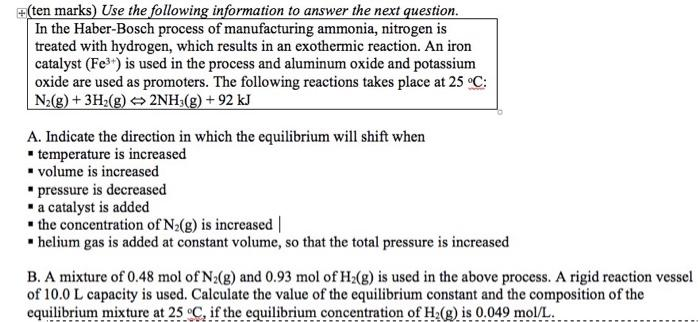### Create an Account

Home / Questions / Use the following information to answer the next question. In the Haber-Bosch process of m...

# Use the following information to answer the next question. In the Haber-Bosch process of manufacturing ammonia, nitrogen is treated with hydrogen, which results in an exothermic reaction. An iron cata

Use the following information to answer the next question. In the Haber-Bosch process of manufacturing ammonia, nitrogen is treated with hydrogen, which results in an exothermic reaction. An iron catalyst (Fe3+) is used in the process and aluminum oxide and potassium oxide are used as promoters. The following reactions takes place at 25°C: N2(g) + 3H2(g) + 2NH3(g) +92 kJ A. Indicate the direction in which the equilibrium will shift when temperature is increased volume is increased pressure is decreased - a catalyst is added - the concentration of N2(g) is increased helium gas is added at constant volume, so that the total pressure is increased B. A mixture of 0.48 mol of N2(g) and 0.93 mol of H2(g) is used in the above process. A rigid reaction vessel of 10.0 L capacity is used. Calculate the value of the equilibrium constant and the composition of the equilibrium mixture at 25°C, if the equilibrium concentration of H2(g) is 0.049 mol/L.May 15 2021 View more View LessSubscribe To Get Solution